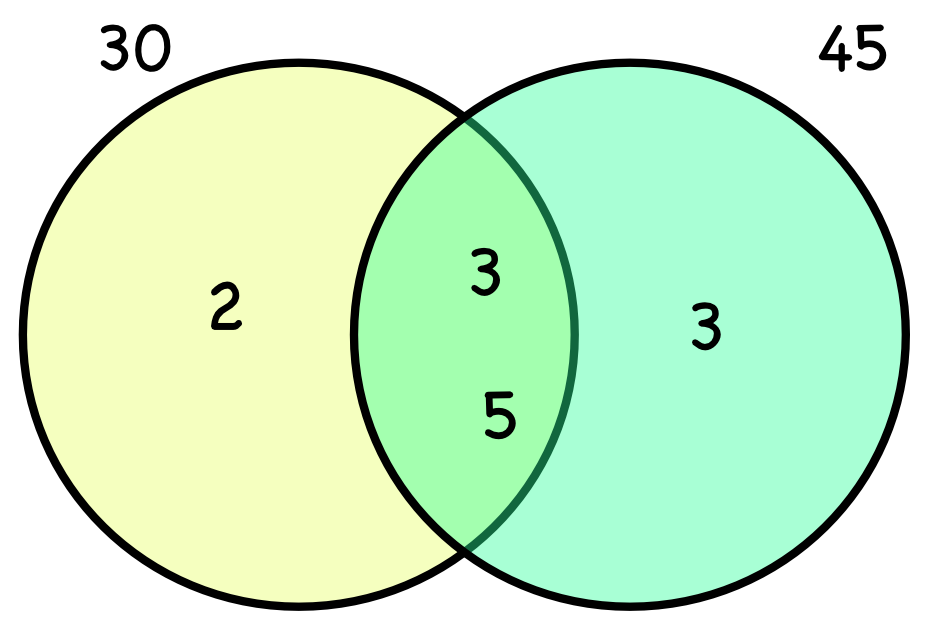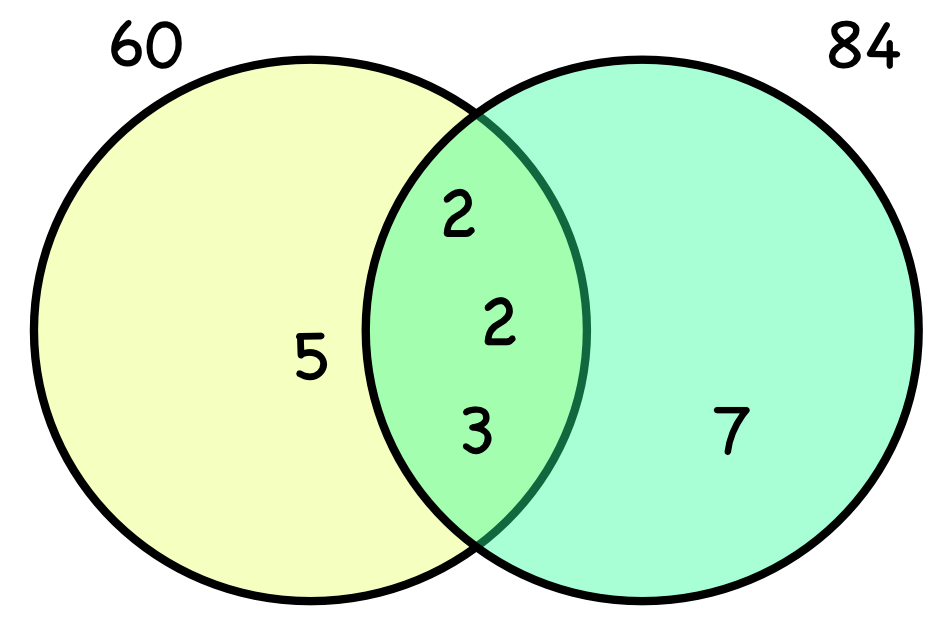Highest Common Factor

## Highest Common Factor

The Highest Common Factor, or HCF, is the largest factor that is shared by two integers. For smaller numbers, the HCF can be determined by writing out a list of factors for each number, and determining the highest number which is on both lists:

The factors of 30 are 1, 2, 3, 5, 6, 10, 15 and 30

The factors of 45 are 1, 3, 5, 9, 15 and 45.

The highest number that is on both lists is 15: this is the Highest Common Factor (or HCF).

For larger numbers, work out the Products of the Primes for both numbers using a prime factor tree. Draw a Venn Diagram, with each set containing the factors for each number. The shared factors (intersection), when multiplied together, will give the HCF. The intersection can be written as factors of 30 ∩ factors of 45.Higher/Extended only:To find the Highest Common Factor of more than two numbers, create a Venn Diagram with a set of prime factors for each number. The intersection of ALL the sets will give the factors for the Highest Common Factor for ALL the numbers.

## Example 1

What is the highest common factor of 60 and 84?

60: 2 x 2 x 3 x 5

84: 2 x 2 x 3 x 7

Factors in both sets are 2, 2 and 3: 2 x 2 x 3 = 12.## Example 2

What is the highest common factor of 63 and 120?

Create a prime factor tree for 63 and 120.

63: 3 x 3 x 7

120: 2 x 2 x 2 x 3 x 5

There is only one factor in both sets: 3